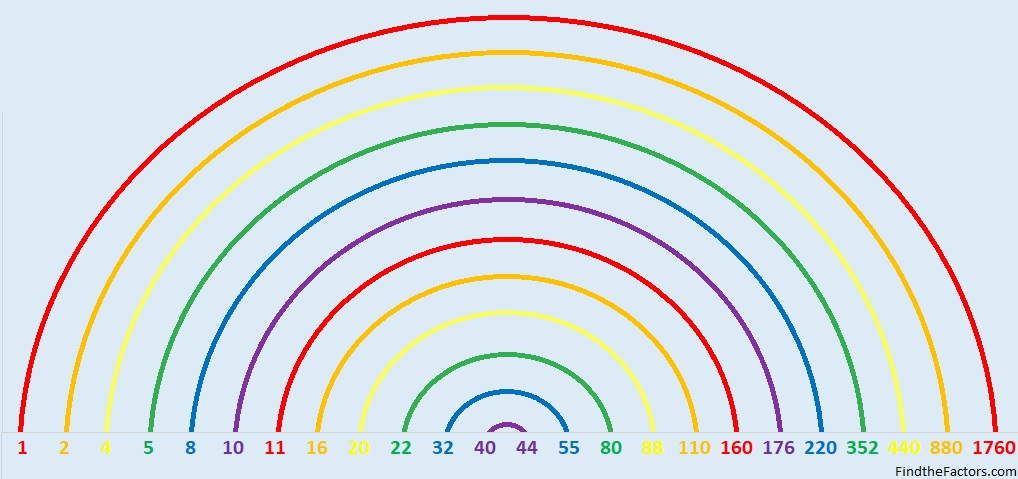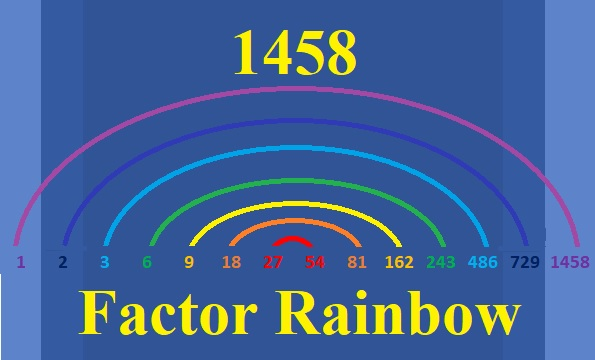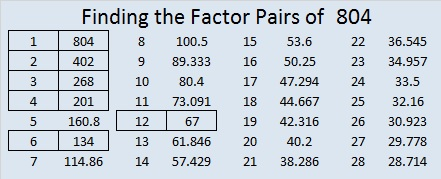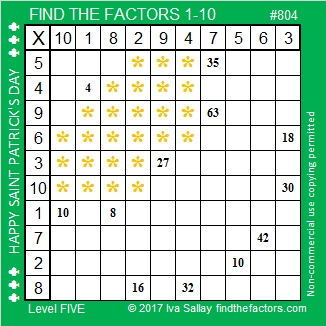# 1760 Pots of Gold and Rainbows

### Today’s Puzzle:

Write the numbers 1 to 12 in the first column and again in the top row so that those numbers are the factors that make the given clues. It’s a level 6, so it won’t be easy. Finding a leprechaun’s pot of gold isn’t easy either. Still, if you can solve this puzzle, then you will have found some real golden nuggets of knowledge.They say there’s a pot of gold at the end of the rainbow. Where’s the rainbow?

### Factors of 1760:

Puzzle number, 1760, has many factors. It makes a very big factor rainbow!• 1760 is a composite number.
• Prime factorization: 1760 = 2 × 2 × 2 × 2 × 2 × 5 × 11, which can be written 1760 = 2⁵ × 5 × 11.
• 1760 has at least one exponent greater than 1 in its prime factorization so √1760 can be simplified. Taking the factor pair from the factor pair table below with the largest square number factor, we get √1760 = (√16)(√110) = 4√110.
• The exponents in the prime factorization are 5, 1, and 1. Adding one to each exponent and multiplying we get (5 + 1)(1 + 1)(1 + 1) = 6 × 2 × 2 = 24. Therefore 1760 has exactly 24 factors.
• The factors of 1760 are outlined with their factor pair partners in the graphic below.### More About the Number 1760:

1760 is the hypotenuse of a Pythagorean triple:
1056-1408-1760 which is (3-4-5) times 352.

1760 is the difference of two squares in eight different ways:
441² – 439² = 1760,
222² – 218² = 1760,
114² – 106² = 1760,
93² – 83² = 1760,
63² – 47² = 1760,
54² – 34² = 1760,
51² – 29² = 1760,  and
42² – 2² = 1760. (That means we are only four numbers away from the next perfect square!)

1760 is palindrome 2102012 in base 3
because 2(3⁶)+1(3⁵)+ 0(3⁴)+2(3³)+0(3²)+1(3¹)+2(3º) = 1760.

# 1458 Tangrams Can Be A Pot of Gold

### A Tangram Puzzle

Tangrams are seven puzzle pieces that can form a square but can also be made into many different people, places, and things. A lot of stress is going on in the world right now, but since tomorrow is Saint Patrick’s Day, we can still find a little pot of gold at the end of the rainbow!

I made this pot of gold on Desmos using points and equations. If you cut it apart, will you be able to put it back together again?What other things can you make from those seven tangram shapes?

And what about that rainbow I mentioned? The number 1458 makes a lovely factor rainbow.

#### A Factor Rainbow for 1458:#### Factors of the number 1458:

• 1458 is a composite number.
• Prime factorization: 1458 = 2 × 3 × 3 × 3 × 3 × 3 × 3, which can be written 1458 = 2 × 3⁶.
• 1458 has at least one exponent greater than 1 in its prime factorization so √1458 can be simplified. Taking the factor pair from the factor pair table below with the largest square number factor, we get √1458 = (√729)(√2) = 27√2
• The exponents in the prime factorization are 1 and 6. Adding one to each exponent and multiplying we get (1 + 1)(6 + 1) = 2 × 7 = 14. Therefore 1458 has exactly 14 factors.
• The factors of 1458 are outlined with their factor pair partners in the graphic below.#### More Facts about the Number 1458:

2 is a prime factor of 1458 exactly one time, so there are NO ways that 1458 can be written as the difference of two squares.

2 and 3 are the only primes appearing in its prime factorization, so 1458 is NEVER the hypotenuse of a Pythagorean triple.

Nevertheless, since there are three different ways that 1458 = 2(a)(b), where a > b, there are three ways that 1458 is a leg in a Pythagorean triple:
1458-531440-531442, calculated from 2(729)(1), 729² – 1², 729² + 1²
1458-59040-59058, calculated from 2(243)(3), 243² – 3², 243² + 3²
1458-6480-6642, calculated from 2(81)(9), 81² – 9², 81² + 9²

Why can’t we get a Pythagorean triple from 1458 = 2(27)(27)? I’m sure you can figure out that one yourself.

# 804 Is There a Pot of Gold at the End of This Rainbow?

Factor Rainbows can be a wonderful way to display the factors of a number. Not only are all the factors listed in order from smallest to greatest, but the factor pairs are joined together with the same color band.

The number 804 has 12 factors so it makes a lovely rainbow with 6 different color bands.Is there a pot of gold at the end of this factor rainbow? I’ll let you decide the answer to that question.Print the puzzles or type the solution on this excel file: 10-factors 801-806

Finding golden nuggets of information about a number might be less difficult than finding pots of gold.

I always begin the painstaking mining process by looking at the factors of the number:

• 804 is a composite number.
• Prime factorization: 804 = 2 x 2 x 3 x 67, which can be written 804 = (2^2) x 3 x 67
• The exponents in the prime factorization are 2, 1, and 1. Adding one to each and multiplying we get (2 + 1)(1 + 1)(1 + 1) = 3 x 2 x 2 = 12. Therefore 804 has exactly 12 factors.
• Factors of 804: 1, 2, 3, 4, 6, 12, 67, 134, 201, 268, 402, 804
• Factor pairs: 804 = 1 x 804, 2 x 402, 3 x 268, 4 x 201, 6 x 134, or 12 x 67
• Taking the factor pair with the largest square number factor, we get √804 = (√4)(√201) = 2√201 ≈ 28.3548937575Finding nuggets of information about the number 804 has been a little difficult and disappointing:

• None of 804’s prime factors can be written as 4N+1, so 804 is NOT the hypotenuse of any Pythagorean triples.
• 804 is NOT a palindrome in base 36 or any base less than that.
• 804 is NOT the sum of any consecutive prime numbers.

Even though I did not find any golden nuggets in those places, I kept looking and finally found a couple of gems about the number 804:

804 can be written as the sum of three squares four different ways, and all of those ways have some definition of double in them:

• 28² + 4² + 2² = 804
• 26² + 8² + 8² = 804
• 22² + 16² + 8² = 804
• 20² + 20² + 2² = 804

OEIS.org also gives us a nugget about the number 804 that may be a bit too heavy for most people to handle: “804 is a value of n for which 2φ(n) = φ(n+1).” That basically means that there are exactly half as many numbers less than 804 that are NOT divisible by its prime factors (2, 3, or 67) as there are numbers less than 805 that are NOT divisible by its prime factors (5, 7, or 23).

I started looking for golden specs about 804 in places that I don’t usually look.

267 + 268 + 269 = 804 so 804 is the sum of 3 consecutive numbers.

As stated before 804 is never the hypotenuse of a Pythagorean triple. However to find all the times it is a leg in a triple will require a lot of labor especially since 804 has so many factors, including 4, and two of its factor pairs have factors where both factors are even.

• 134 × 6 is an even factor pair, so (134 + 6)/2 = 70, and (134-6)/2 = 64. Thus 804 = 134·6 = (70 + 64)(70 – 64) = 70² – 64² .
• 402 × 2 is another even factor pair, so (402 + 2)/2 = 202, and (402 – 2)/2 = 200. Thus 804 = 402·2 = (200 + 2)(200 – 2) = 202² – 200²
• Likewise odd or even sets of factor pairs of any of 804’s factors can also be used to find Pythagorean triples.

So to find all Pythagorean triples that contain the number 804, we will have to find all the times 804 satisfies one of these FOUR conditions:

1. 804 = 2k(a)(b) so that 804 is in the triple 2k(a)(b), k(a² – b²), k(a + b²) OR the triple k(a² – b²), 2k(a)(b), k(a + b²).
2. 804 = 2(a)(b) so that 804 is in the triple 2(a)(b), a² – b², a + b² OR the triple a² – b², 2(a)(b), a + b².
3. 804 = a² – b² so that 804 is in the triple a² – b², 2(a)(b), a + b² OR the triple 2(a)(b), a² – b², a + b².
4. 804 = k(a² – b²) so that 804 is in the triple k(a² – b²), 2k(a)(b), k(a + b²) OR the triple 2k(a)(b), k(a² – b²), k(a + b²).

Let the mining process begin! I’ll list the triples with the shortest legs first and color code each triple according to the condition I used.

• 335-804-871 which used 804 = 2·67(3)(2) to make a triple that is 5-12-13 times 67
• 603-804-1005 which used 804 = 2·201(2)(1) to make a triple that is 3-4-5 times 201
• 804-1072-1340 which used 804 = 268(2² – 1²) to make a triple that  is 3-4-5 times 268
• 804-2345-2479 which used 804 = 2·67(6)(1) to make a triple that is 12-35-37 times 67
• 804-4453-4525, which used 804 = 2(6)(67)
• 804-8960-8996, which used 804 = 70² – 64² or 804 = 4(35² – 32²) to make a triple that is 201-2240-2249 times
• 804-17947-17965, which used 804 = 2(134)(3)
• 804-26928-26940 which used 804 = 12(34² – 33²) to make a triple that is 67-2244-2245 times 12
• 804-40397-40405, which used 804 = 2(201)(2)
• 804-53865-53871 which used 804 = 2·3(134)(1) to make a triple that is 268-17955-17957 times 3
• 804-80800-80804 which used 804 = 202² – 200²  or 804 = 4(101² – 100²) to make a triple that is 201-20200-20201 times 4
• 804-161603-161605, a primitive Pythagorean triple, that used 804 = 2(402)(1)

If you look for a pot of gold at the end of a rainbow, you’re bound to be disappointed. Science/How Stuff Works just had to crush dreams and dispel 10 Myths About Rainbows. Unfortunately a pot of gold being at the rainbow’s end is included on that list. Still I suppose we could still put every golden spec or nugget about 804 into a little pot and call it a pot of gold.

Or if you are as clever and quick as a leprechaun, perhaps you will consider finding Pythagorean triples to be like finding pots of gold.